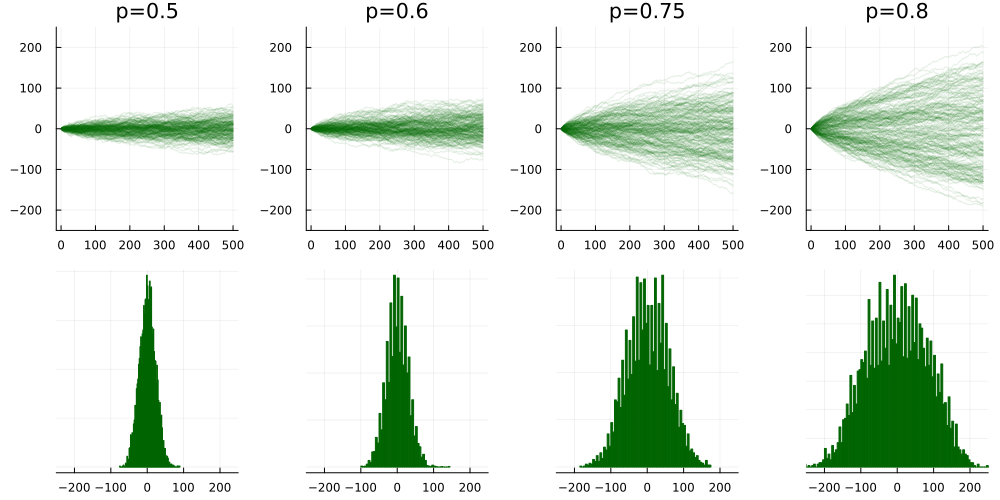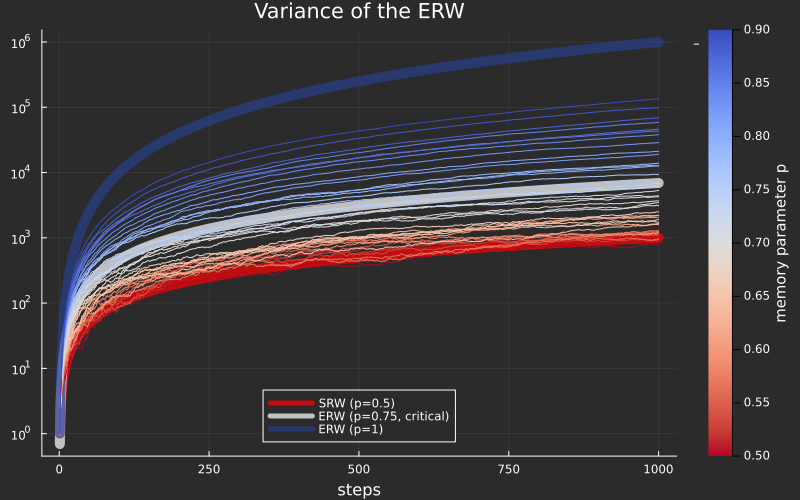# 🐘 The Elephant Random Walk

May 2023

In the Simple Random Walk (ERW), a walker moves in random directions; all of her steps are independent. The variance of the displacement of a random walker after $n$ steps typically grows linearly with $n$: for example in the 1d symmetric random walk defined by

$\mathrm{SRW}_n = S_1 + \dotsb + S_n$

with $\mathbf{P}(S_n =\pm 1) = 1/2$, one has $\mathrm{Var}(\mathrm{SRW}_n) = n$. This behaviour is called diffusive, because it is a discrete analog of heat dissipation.

The introduction of long-term memory in Random Walks breaks this diffusive behaviour. In the Simple Random Walk, the walker takes a random step independently of its former moves, oblivious of her past; but instead, she could remember one of her previous steps and reproduce it, just like Elephants whose memory is said to be surprisingly vast. The Elephant Randow Walk (ERW) has been thoroughly studied since its introduction in the paper Elephants can always remember by Schütz and Trimper, both for its mathematical tractability and rich behaviour.

Here is a description of the Elephant Randow Walk (ERW) with memory parameter $p$.

• The Elephant starts at 0 and takes a first step $S_1=+1$ with initial probability $p_0$ or $S_1 = -1$ with probability $1-p_0$; in fact $p_0$ will not have any influence whatsoever.

• Then, at time $n$, the Elephant randomly remembers one of the steps she took in the past; each of the former steps has the same chance to be remembered. Then, with probability $p$, she reproduces this step; otherwise she goes the opposite way.

Formally, we set $\mathrm{ERW}_0=0$. The first step is $\mathrm{ERW}_1 = \pm 1$ with some probability $p_0$.

At step $k$, let $U_k \sim \mathrm{Uniform}(\{1, \dotsc, k\})$ be the « remembered past step » and let $\varepsilon_k$ be whether the elephant goes in the same or opposite direction, that is $\mathbf{P}(\varepsilon_k = 1) = p$ and $\mathbf{P}(\varepsilon_k = -1) = 1-p$.

The $n+1$-th step is $\mathrm{ERW}_{n+1} = \mathrm{ERW}_n + S_{n+1}$, with the last step $S_{n+1} = \varepsilon_n S_{U_n}$.When the memory parameter $p$ is close to $1$ (full memory), the Elephant tends to reproduce always the same steps, thus going way further than the Simple Randow Walker corresponding to $p=1/2$, and the histogram of its final position is less concentrated.

The goal of this note is to explain the appearance of a sudden and surprising transition, happening at the critical value $p=3/4$: the ERW is still diffusive for $p<3/4$, and becomes super-diffusive if $p>3/4$. It all boils down to computing the variance.

## Computing the variance

When the initial step has a probability $p_0=1/2$ to jump left or right, the whole ERW is symmetric, hence centered. Its variance $V_n = \mathbf{E}[W_n^2]$ solves the following recursion.

$V_1 = 1, \qquad\qquad V_{n+1} = 1 + \left(1 + \frac{4p-2}{n}\right)V_n.$

Proof. We note $\mathscr{F}_n$ the sigma-algebra generated by the jumps up to time $n$. We have

$V_{n+1}=\mathbf{E}[W_{n+1}^2] = \mathbf{E}[W_n^2 + 2S_{n+1}W_n + S_{n+1}^2]=V_n + 1 + 2\mathbf{E}[W_n\mathbf{E}[S_{n+1}\mid \mathscr{F}_n]].$

Conditionnally on $\mathscr{F}_n$, the last jump $S_{n+1}$ is distributed as a Rademacher random variable with a certain parameter $p_n$. Let $N_n$ denote the number of +1 jumps before $n$; then $p_n = pN_n/n + (1-p)(n-N_n)/n$. Since $N_n = (n+W_n)/2$, we obtain

$p_n = \frac{1}{n}\left(p\frac{n+W_n}{2} + q\frac{n-W_n}{2}\right) = \frac{n+(p-q)W_n}{2n}$

where $q=1-p$. The expectation of a Rademacher random variable with parameter $p_n$ is $p_n - (1-p_n) = 2p_n -1$, hence

$\mathbf{E}[S_{n+1}\mid \mathscr{F}_n] = (p-q)\frac{W_n}{n}$

which gives (2) when plugged back in the first expression.

The variance of the Elephant Randow Walk has the following explicit expression depending on $\alpha = 4p-2$: $\mathrm{Var}(W_n) = \frac{\Gamma(\alpha+n)}{\Gamma(\alpha)\Gamma(n)} \int_0^1 (1-x)^{(\alpha-1)-1} (1-x^n)\mathrm{d}x.$

The proof is postponed to later. This expression might not be very informative at first sight, but its large-$n$ asymptotics are easily understood and unveil a qualitative change in the behaviour of the ERW at the critical value $p=3/4$. By symmetry we restrict to $p>1/2$.

Diffusive case: if $p<3/4$,

$V_n \sim \frac{n}{3-4p}.$

Critical case: if $p=3/4$,

$V_n \sim n\log(n).$

Super-diffusive case: if $p>3/4$,

$V_n \sim \frac{n\times n^{4p-3}}{(4p-3)\Gamma(4p-2)}$

Proof. Let us note $I_n(\alpha)$ the integral in (6). Its behaviour depends on wheter $\alpha-1 = 4p-3$ is positive or negative. We'll make use of Stirling's formula, $\Gamma(x) \sim x^{x}e^{-x}\sqrt{2\pi x}$, which ensures that

$\frac{\Gamma(\alpha+n)}{\Gamma(\alpha)\Gamma(n)} \sim \frac{(\alpha+n)^\alpha}{\Gamma(\alpha)}.$

Now,

• If $\alpha-1>0$ then $I_n(\alpha)\sim \int_0^1 (1-x)^{\alpha-2}dx = (\alpha-1)^{-1}$;

• If $\alpha=1$ then $I_n(\alpha)=1+1/2+1/3 +\dotsb + 1/n\sim \log(n)$;

• If $\alpha-1<0$ then $I_n(\alpha)\sim \Gamma(\alpha)n^{-\alpha}n/(1-\alpha)$

Here is a log-plot of the empirical variance of 100 runs of Elephant Randow Walks with various memory parameters $p$ ranging between $0.5$ and $0.9$.The white line marks the transition at $p=3/4$, between a diffusive behaviour and a super-diffusive behaviour. The red line represents the Simple Random Walk with a variance of $n$.

## Proof of (6): exact computation of the variance $V_n$

Recursions like (2) are easy to solve. Let us note $\alpha = 2(p-q)$ and $x_n = (n + \alpha)/n$ so that $V_{n+1} = 1 + x_n V_n$. Define

$c_n = x_{n-1}x_{n-2}\times\dotsm\times x_2x_1$

and $V'_n = V_n/c_n$ –- we chose $c_n$ so that $c_nx_n = c_{n+1}$. Then, (2) becomes

$V'_{n+1} = \frac{1}{c_{n+1}} + \frac{V_n}{c_{n}}\frac{c_n x_n}{c_{n+1}} = \frac{1}{c_{n+1}} + V'_n.$

By a telescoping sum and using $V'_1 = 1$,

$V'_{n} = \frac{1}{c_{n}} + \frac{1}{c_{n-1}} + \dotsb + \frac{1}{c_2} + 1.$

Now we see that

$c_{n+1} = x_n x_{n-1}\times \dotsm \times x_1 = \frac{(\alpha+n)(\alpha+n-1)\times \dotsm \times (2+\alpha)(1+\alpha)}{n(n-1)\times \dotsm \times 2\times 1}= \frac{\Gamma(\alpha+n)}{\Gamma(n)\Gamma(\alpha+1)}$

hence the exact formula

\begin{aligned}V'_{n} &= \sum_{k=1}^n \frac{\Gamma(k)\Gamma(1+\alpha)}{\Gamma(k+\alpha)}. \end{aligned}

Now since $V_n = c_n V'_n$, we get

\begin{aligned} V_n = \frac{\Gamma(\alpha+n)}{\Gamma(\alpha+1)\Gamma(n)}\sum_{k=1}^n\frac{\Gamma(k)\Gamma(1+\alpha)}{\Gamma(k+\alpha)} &= \frac{\Gamma(\alpha+n)}{\Gamma(\alpha)\Gamma(n)}\sum_{k=1}^n\frac{\Gamma(k)\Gamma(\alpha)}{\Gamma(k+\alpha)}. \end{aligned}

This expression can be further simplified using the $\Gamma-\beta$ formula, valid as soon as $\alpha>0$ (this is the case when $p>1/2$).

\begin{aligned} V_n&=\frac{\Gamma(\alpha+n)}{\Gamma(\alpha)\Gamma(n)}\sum_{k=1}^n \int_0^1 x^{k-1}(1-x)^{\alpha-1}dx\\ &=\frac{\Gamma(\alpha+n)}{\Gamma(\alpha)\Gamma(n)} \int_0^1 (1-x)^{\alpha-1} \frac{1-x^n}{1-x}dx\\ &=\frac{\Gamma(\alpha+n)}{\Gamma(\alpha)\Gamma(n)} \int_0^1 (1-x)^{\alpha-2} (1-x^n)dx. \end{aligned}

## Conclusion and references

Many aspects of the ERW are now well understood. Its introduction in the papers Elephants can always remember and Anomalous dynamics… was mostly motivated by the physics of non-Markovian diffusions; they even formulated the continuous-time version with a non-diffusive Fokker-Planck equation. The effect of the type of memory on diffusive properties was studied in this paper.

Mathematically, the functional limit for the ERW was proved in a very elegant way in this paper – it appears that the ERW converges towards a continuous Gaussian process with a particular covariance kernel. The use of martingale theory turned out to be very powerful and allows for an efficient identification of the scaling limits of the ERW, see Bercu's paper. Multi-dimensional versions were studied in this paper.